# Which is easier?

Assessing your students’ current knowledge of, and comfort with, a particular topic is important before trying to build on that topic. It is especially important if, like I’ve done on occasion in recent years, you’re supply teaching and find yourself in front of classes that you don’t know well, if at all.

Rather than work through a set of routine questions to try and gauge their current mathematical level, I’ve found an approach that often does a good job of (1) hooking students and (2) encouraging them to think hard. The approach involves presenting them with two questions—not necessarily to complete, but to compare. Specifically, they must then decide which of questions they think they’d find easier (or quicker) to answer. Importantly, they need to give a reason why they think they could do one question more easily/ quickly than the other. Sometimes I’d get students to write their explanations so I could glance at them while circulating the room. More often, I’d get them to discuss in pairs—whilst I’d do my best to eavesdrop.

A neat thing about these is that they’re so quick to come up with and to handwrite on the board. Here are a few typed-up examples of such “which is easier?” tasks, along with some of my thoughts on what I was hoping to dig into with each one.

## A few examples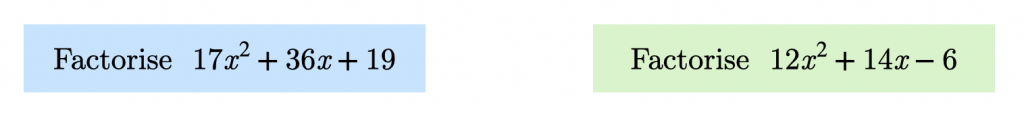I like to encourage students to factorise quadratics by inspection—though this is perhaps better described as strategic trial and error. My experience with students at a range of schools is that the grouping method (also known as the ac method, or “split the middle term” method) is most widely used. While I wouldn’t wish to “undo” what they’ve been taught, I do try to get students to at least try to consider whether the factorisation is “easy” to spot. In this case, I find the first one easier: the prime $$a$$ term and positive $$b$$ and $$c$$ tells us we’ll have something of the form $$(17x+p)(x+q)$$. The prime $$c$$ term tells us that $$p$$ and $$q$$ must be 1 and 19—though not necessarily in that order. A quick check is all that would be needed to complete the question. The other expression, with its composite $$a$$ and $$c$$ terms is likely to take longer, despite each coefficient being smaller and perhaps more innocuous-looking.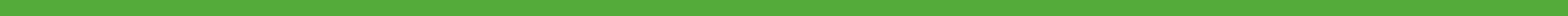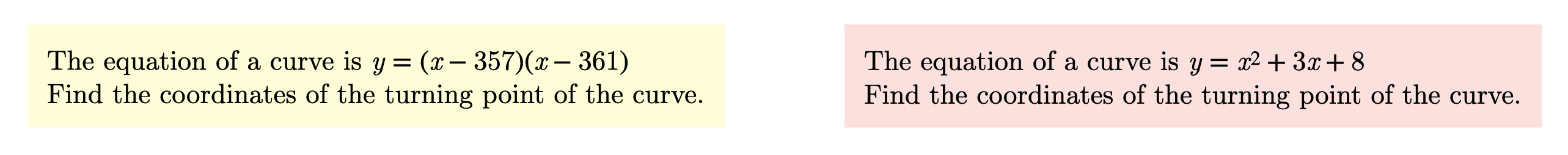Often, students seem to build an inflexible, “flow-chart” type approach to maths. I spoke about this at my MathsConf27 presentation, titled Mathematical straitjackets. Here, for example, some students will read “turning point” and think “I have to complete the square.” This is not the case, however. With a strong, interconnected understanding of quadratics (graphs, roots, factorisation, vertex form etc.), we can see that the first equation has roots $$357$$ and $$361$$. This means that the parabola’s line of symmetry is $$x = 359$$ and that the $$x$$-coordinate of the turning point is $$359$$. Substituting $$359$$ into $$y = (x-357)(x-361)$$, we see $$y=-4$$ so the turning point here is $$(359,-4)$$. To my mind, this is less of a faff than trying to rewrite $$y=x^2+3x+8$$ in vertex form.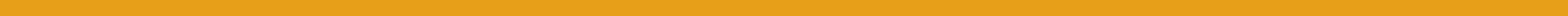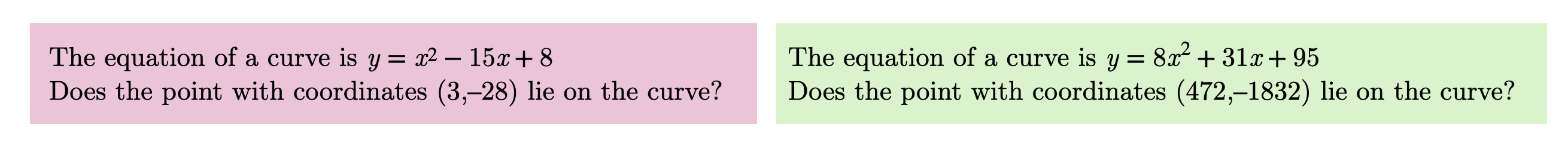Here, I wanted to give students a reminder to keep the bigger picture in mind. The positive coefficients in the second equation mean that for a point on the curve where x is positive, y must also be positive. So we can immediately state that $$(472,-1832)$$ is not on the curve.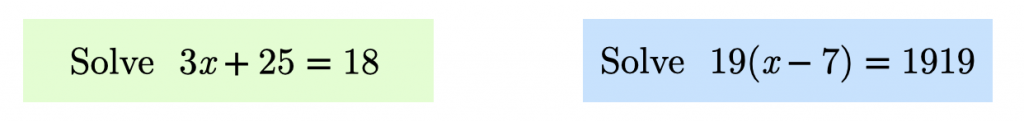Some students demonstrate a flow-chart mentality again, by either thinking they have to, or choosing, to expand $$19(x-7)$$ first. In this example, dividing both sides by 19 and then adding 7 to both, to my mind, is as easy as to do as any of the calculations needed to solve the other equation.I’ve encountered a handful of students who have been taught (usually by a parent, rather than their teacher) to always convert mixed numbers into improper fractions before performing arithmetic. It’s not wrong to do this per se, but it can be inefficient.

You’ve probably noticed that in all these examples, the question with the trickier-looking numbers is always the “easier” one—at least to someone with sufficient mathematical expertise. This leads us on to a possible objection to such tasks:

## But surely students will eventually spot that the questions with “trickier” numbers are the easier ones?

This has been my experience—it doesn’t escape students’ notice that I always end up claiming that the harder-looking questions are the ones I would find easier. This is, to borrow a phrase, is a feature, not a bug. Students might see a pair of questions, knowing full well which one I’m going to claim is easier, but not see why it is easier. This kind of situation—where they know what the “answer” is—encourages students to think hard about the why the answer is what it is. They’re not getting bogged down trying to work out the answers to either of the questions; they’re focussing on important aspects of the questions to decide which is easier. Remember, the goal with these isn’t just for students to identify which question is easier—they also have to give reasons for their choice.

Furthermore, we can always keep them on their toes with a double bluff. Whilst the nastier-looking question is the easier one of the first pair, it is (for once) the easier-looking question of the second pair that is genuinely easier.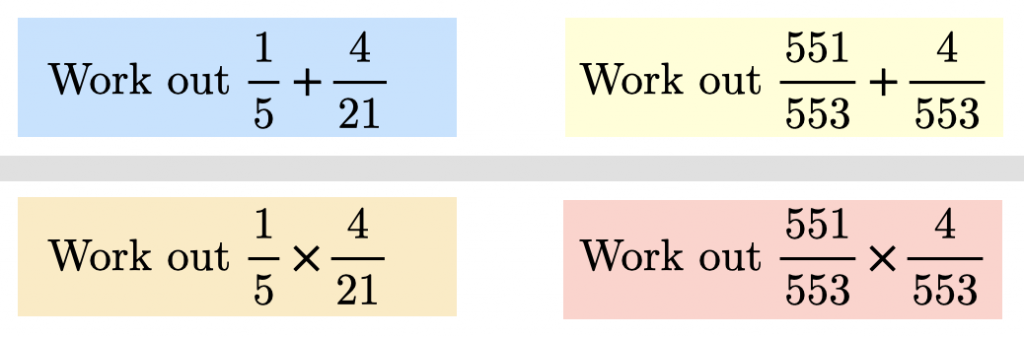## Extending the idea

I’ve only given examples at KS3-4, but they have a lot of potential at KS5: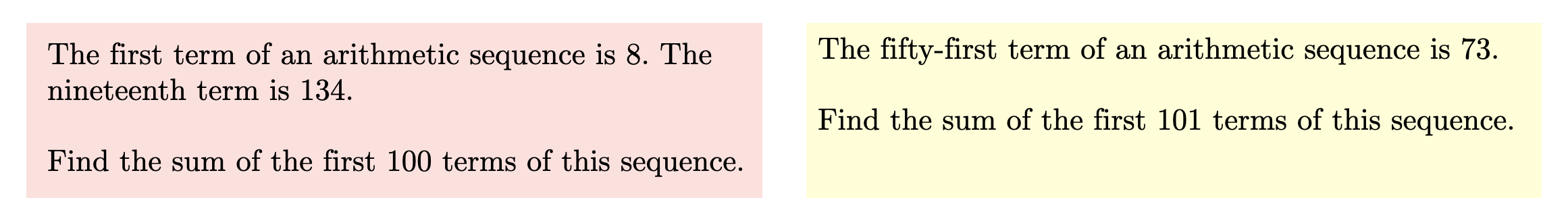Students with only a formulaic appreciation of summing terms of an arithmetic series would almost certainly prefer the question on the left. They can quickly find $$d$$ and substitute this and the given values of $$a$$ and $$n$$ into the formula $$S_n=\frac{1}{2}n\left(2a+d(n-1)\right)$$. With an appreciation of the structure of arithmetic sequences, we might see that the sum is simply the number of terms multiplied by the median term. In the second question, we are immediately given the number of terms ($$101$$) and the median term ($$73$$).Of course, these tasks don’t need to exist in this particular format, with two questions being compared. Here is one where students have to decide which of three questions is easiest. This task did require a little more thought and preparation on my part than any of those above, however.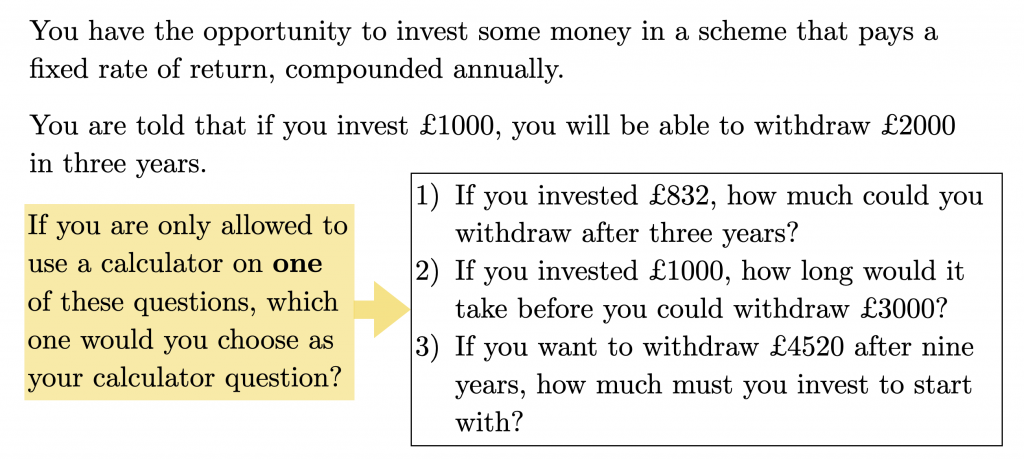In this case, question 2, with the “nicest” numbers (again), is the “hardest” one—i.e. the one I would want a calculator for. The others can be worked out mentally by noting that your money doubles every three years.